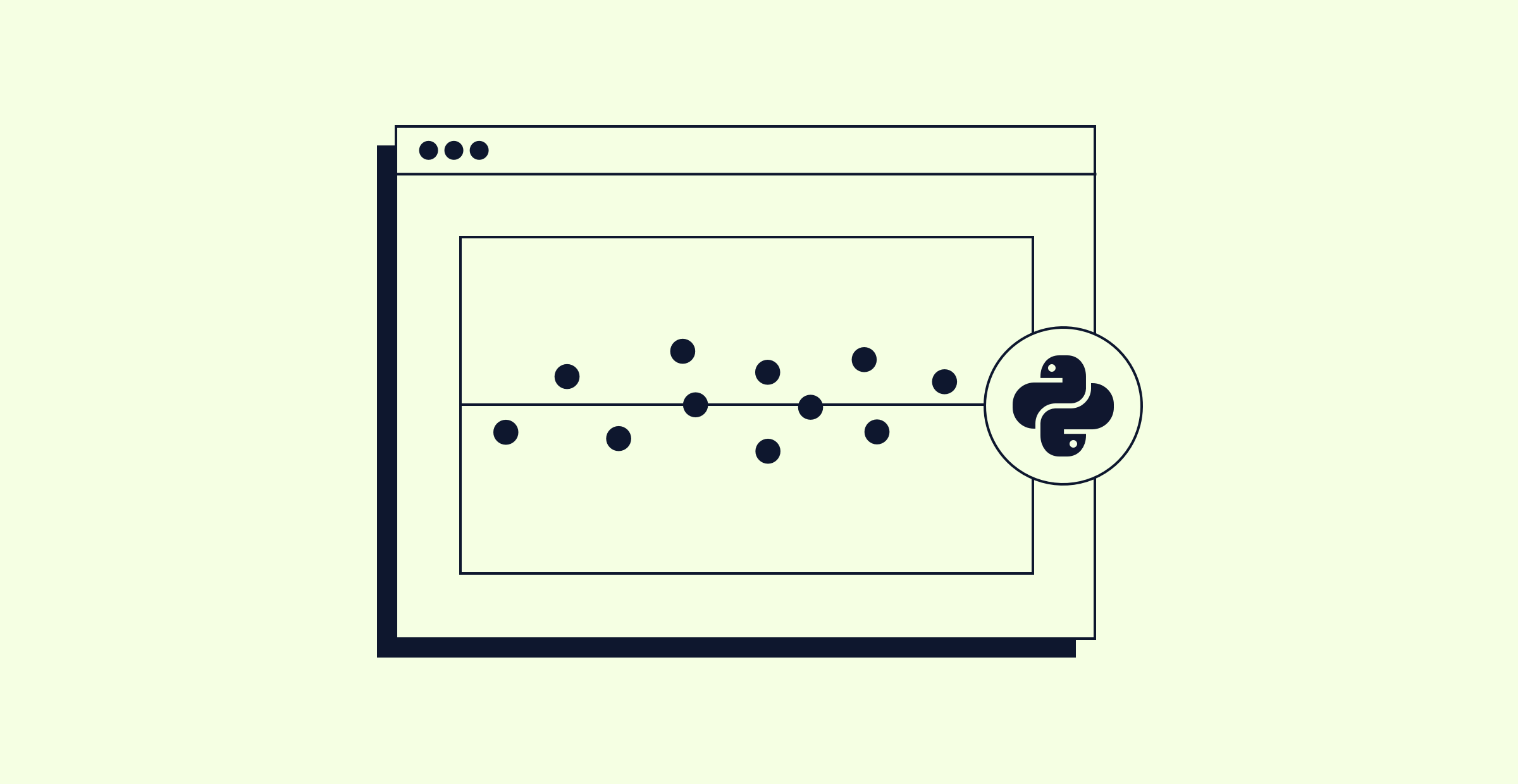# Level Up: Linear Regression in Python - Part 3In the third lesson of the series, we'll implement our first linear regression model with multiple predictors (this is called "multiple linear regression"). As an example, we'll use a simulated dataset to predict student quiz scores. In the process, we'll again practice our graphing and Python skills.

Here are some Stack Overflow questions related to the work we did in today's session:

If you want to ask any questions or provide feedback on the lesson, you are welcome to leave a comment on the YouTube recording of this lesson. If you’d like to watch a session live, follow the Codecademy YouTube channel. We'll be live again on Tuesday, June 8 at 11am EDT to discuss the matrix representation of the linear regression problem. You can join that session here.

Finally, if you want even more linear regression content, you can sign up for the Linear Regression in Python interactive course this series was based on. This course was developed by Sophie and has many more quizzes, projects, and helpful nuggets that we can’t fit into our streams!